# TSP 问题

TSP 问题（Traveling Salesman Problem）又译为旅行推销员问题、货郎担问题，是数学领域中著名问题之一。假设有一个旅行商人要拜访 n 个城市，他必须选择所要走的路径，路径的限制是每个城市只能拜访一次，而且最后要回到原来出发的城市。路径的选择目标是要求得的路径路程为所有路径之中的最小值。——百度百科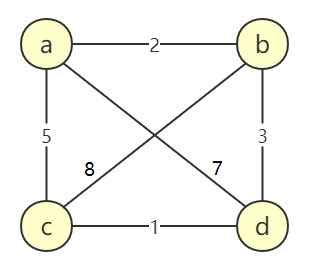1 a->b->c->d->a 18
2 a->b->d->c->a 11
3 a->c->b->d->a 23
4 a->c->d->b->a 11
5 a->d->b->c->a 23
6 a->d->c->b->a 18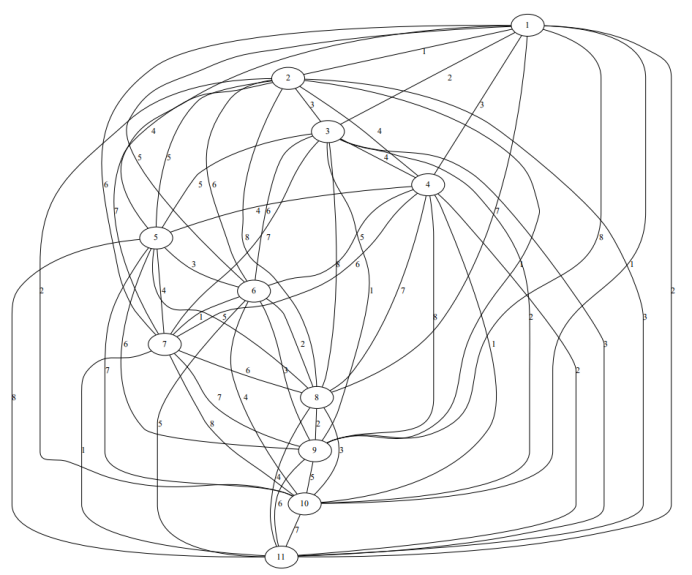# 实验程序编写

## 图结构体定义

TSP 是个 NP 完全问题，我们需要图结构来进行存储。我选择邻接矩阵存储城市拓扑图，定义的图结构体如下。

typedef struct    //图的定义
{
int edges[MAXV][MAXV];    //邻接矩阵
int n;    //顶点数
} MGraph;


## 城市拓扑的建立

MGraph CreateMGraph(int num)    //建图
{
MGraph topography;

for (int i = 1; i <= num; i++)
{
for (int j = 1; j <= num; j++)
{
topography.edges[i][j] = 0;
}
}
for (int i = 1; i <= num; i++)
{
for (int j = i + 1; j <= num; j++)
{
printf("城市%d和城市%d之间的距离为:",i,j);
cin >> topography.edges[i][j];
topography.edges[j][i] = topography.edges[i][j];
}
}
topography.n = num;
}


## DFS

void DFS(int new_point, int cities_visited, int &path_index)    //深度遍历
{
count++;
if (cities_visited == topography.n)    //所有城市都走一遍
{
path[path_index][cities_visited] = new_point;
path[path_index][cities_visited + 1] = start_point;    //回到出发点
for(int i = 1; i <= topography.n; i++)
{
path[path_index + 1][i] = path[path_index][i];    //下一条路径拷贝上一条
}
path_index++;
}
else
{
for (int i = 1; i <= topography.n; i++)
{
if (visited[i] == 0)
{
visited[i] = 1;
path[path_index][cities_visited] = new_point;
DFS(i, cities_visited + 1, path_index);
visited[i] = 0;    //回溯到上一城市
}
}
}
return;
}


## 主函数

int main()
{
int cities_num = 0;    //城市数量
int path_num = 1;    //路径数
int cities_visited = 1;    //已访问城市数
int path_index = 1;    //已获取的路径数
int min_path = 0;
int min_sum = 9999999;
int sum;

cout << "城市数量为：";
cin >> cities_num;
//建图
topography = CreateMGraph(cities_num);
for(int i = cities_num - 1; i > 1; i--)
{
path_num *= i;
}
//初始化访问状态
for(int i = 1; i <= topography.n; i++)
{
visited[i] = 0;
}
//出发
cout << "从哪个城市出发：";
cin >> start_point;
visited[start_point] = 1;
//获取所有路径
DFS(start_point, cities_visited, path_index);
//得出最短路径
ofstream outfile;
outfile.open("11.txt");
for (int i = 1; i < path_index; i++)
{
sum = 0;
outfile << "路径" << i << ":";
for (int j = 1; j <= cities_num; j++)
{
sum += topography.edges[ path[i][j] ][ path[i][j + 1] ];
}
if(sum < min_sum)
{
min_sum = sum;
min_path = i;
}
}
cout << "\n最短路径为路径" << min_path << ":";
for (int j = 1; j <= cities_num; j++)
{
cout << path[min_path][j] << " -> ";
outfile << path[min_path][j] << " -> ";
sum += topography.edges[ path[min_path][j] ][ path[min_path][j + 1] ];
}
cout << path[min_path][cities_num + 1] << endl;
cout << "最短路径长度为:" << min_sum << endl;
cout << "DFS 次数为:" << count;
return 0;
}


# 获取实验数据

4 6 16 1
5 24 65 2
6 120 326 7
7 720 1957 45
8 5070 13700 348
9 40320 109601 3050
10 362880 986410 30260
11 3628800 9864101 330943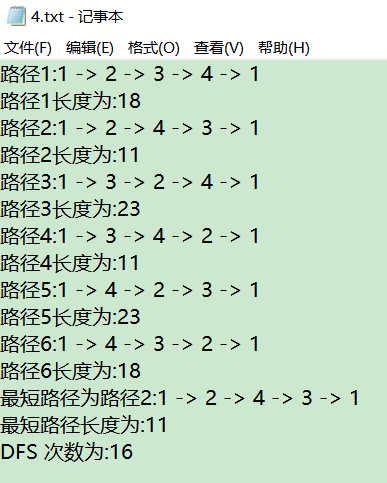# 实验数据分析

## 路线数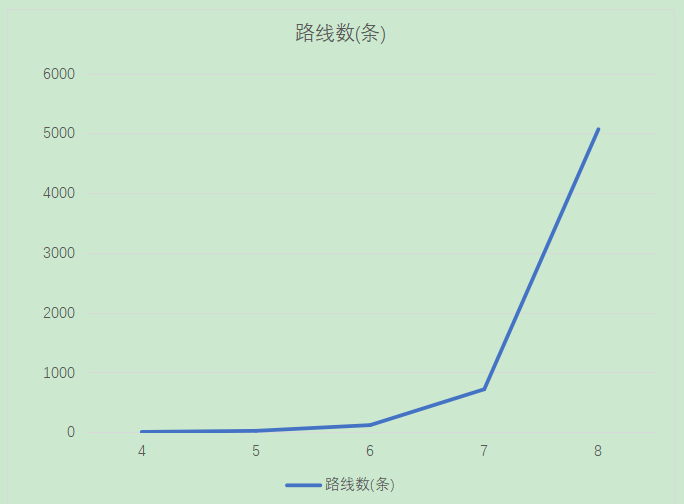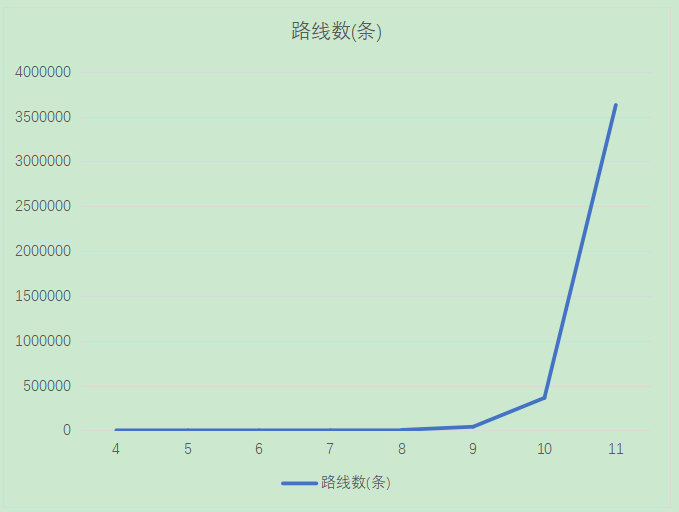## DFS 次数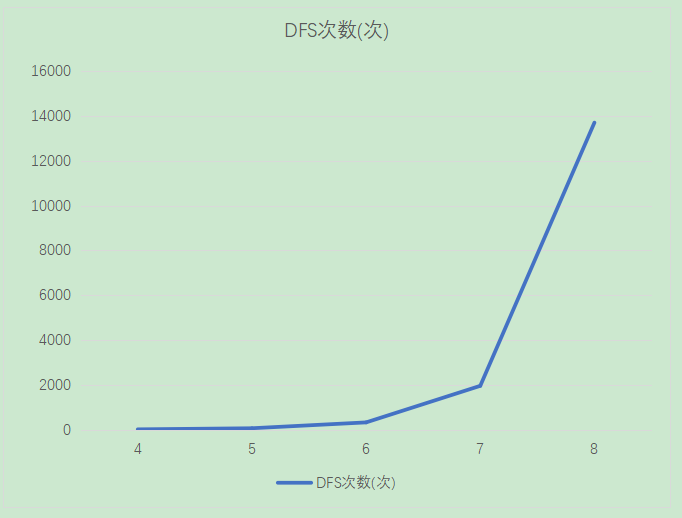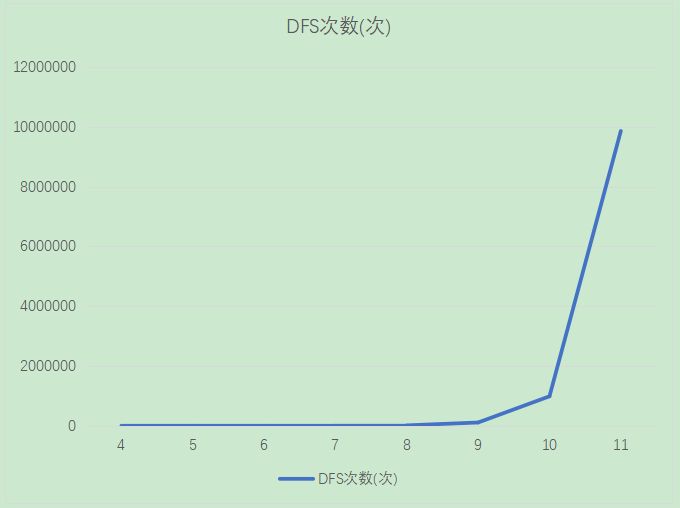## 数据文件大小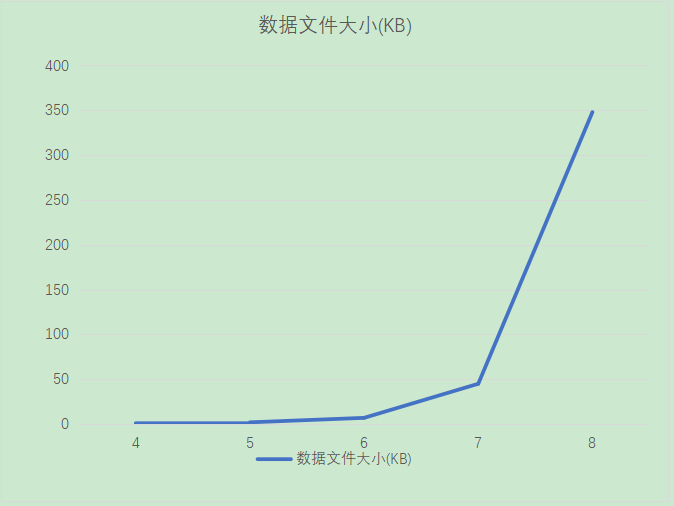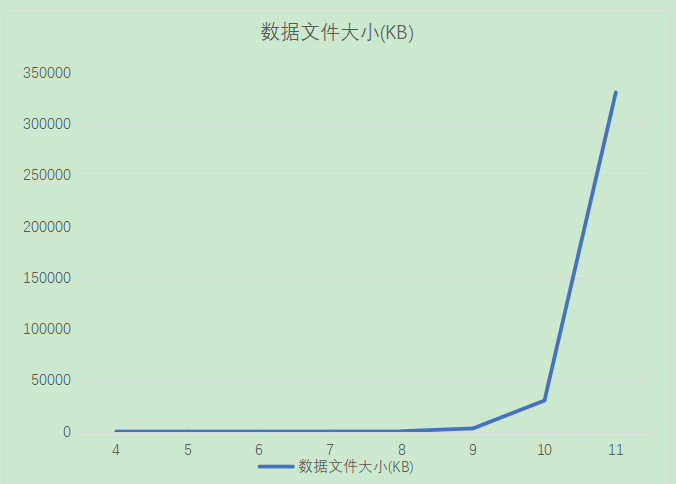# 参考资料

《数据结构(C语言版|第二版)》—— 严蔚敏 李冬梅 吴伟民 编著，人民邮电出版社
《算法设计与分析(第二版)》——王红梅，胡明 编著，清华大学出版社
Graphviz 安装并使用 (Python)
C++文件和流

posted @ 2020-10-26 21:34  乌漆WhiteMoon  阅读(190)  评论(0编辑  收藏